# Algebraic number

(diff) ← Older revision | Latest revision (diff) | Newer revision → (diff)

A complex (sometimes, real) number that is a root of a polynomial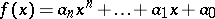(1)

with rational coefficients, not all of which are zero. Ifis an algebraic number, then, among all polynomials with rational coefficients andas a root, there exists a unique polynomial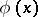of lowest degree with leading coefficient equal to one, which is therefore irreducible (cf. Irreducible polynomial). It is called the irreducible, or minimal, polynomial of the algebraic number. The degreeof the minimal polynomialis also called the degree of the algebraic number. The existence of irreducible polynomials of any degreeimplies the existence of algebraic numbers of degree. All rational numbers, and only such numbers, are algebraic numbers of the first degree. The numberis an algebraic number of the second degree, since it is a root of the polynomial, while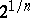, whereis any positive integer, is an algebraic number of degree, being a root of the irreducible polynomial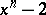.

The roots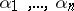of the irreducible polynomial are called the conjugates of, and are also algebraic numbers of degree. All numbers conjugate withare distinct. Apart from its degree, another important characteristic of an algebraic number is its height, which is the analogue of the denominator of a rational fraction. The height of an algebraic numberis the greatest absolute value of the coefficients of the irreducible and primitive polynomial with integral rational coefficients that hasas a root (cf. Primitive polynomial). The sum, the difference, the product and the quotient of two algebraic numbers (except for division by zero) are algebraic numbers; this means that the set of all algebraic numbers is a field. A root of a polynomial with algebraic coefficients is an algebraic number.

An algebraic number is called an algebraic integer if all the coefficients of its minimal polynomial are rational integers. For instance,and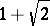are algebraic integers, being roots of the polynomialsand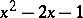.

The concept of an algebraic integer is a generalization of the concept of a rational integer (a rational integeris an algebraic integer, being the root of the polynomial). Many properties of rational integers are also displayed by algebraic integers. Thus, the algebraic integers form a ring; on the other hand, the real algebraic integers form an everywhere-dense set in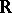, while the rational integers form a discrete set. It was shown in 1872 by G. Cantor that the set of all algebraic numbers is denumerable, and this implied the existence of transcendental numbers (cf. Transcendental number).

A root of any (not necessarily irreducible) polynomial with rational integer coefficients and leading coefficient one is an algebraic integer. Moreover, a root of a polynomial with algebraic integer coefficients and with leading coefficient one is an algebraic integer. In particular the-th degree root of an algebraic integer is an algebraic integer. For any algebraic numberthere exists a positive integersuch thatis an algebraic integer (in analogy with rational numbers). The smallest possible numberis the modulus of the leading coefficient of the irreducible primitive polynomial with rational integer coefficients that hasas a root. All conjugates of an algebraic integer are also algebraic integers.

One says that an algebraic integeris divisible by an algebraic integer,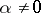, if there exists an algebraic integersuch that. Many divisibility characteristics of rational integers also hold for algebraic integers.

An algebraic integeris called an algebraic unit (or unit for short) if it is a divisor of 1, that is, if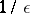is an algebraic integer. A unit is a divisor of any algebraic integer. The inverse of a unit is a unit; the numbers conjugate to a unit are units; all divisors of a unit are units; a product of a finite number of units is a unit. An algebraic integer is a unit if and only if the product of all its conjugates is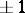. The-th roots of unity are units, each one having modulus 1. There exists an infinite set of other units which do not have modulus 1. For instance, the numbers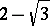and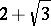are units, being the roots of the polynomial. Moreover, their powers constitute units which may be arbitrary large or small. The field of rational numbers contains only two units —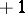and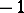.

Two algebraic integers are called associated if they differ by a factor which is a unit. There is another important difference between the ring of algebraic integers and the ring of rational integers. The concept of an irreducible integer (in analogy to a prime number) cannot be introduced into the former. This may be seen from the fact that a root of an algebraic integer is an algebraic integer. The concept of an irreducible number (apart from the class of associated numbers) can be introduced in certain subfields of the field of all algebraic numbers, the so-called algebraic number fields. It turns out, however, that the decomposition of an algebraic integer into irreducible factors is not always unique.

Algebraic numbers cannot be very closely approximated by rational and algebraic numbers (Liouville's theorem). It is this fact which led in 1844 to a proof of the existence of transcendental numbers. The problem of approximation of algebraic numbers by rational numbers is one of the more difficult problems in number theory; attempts to solve it yielded very important results, including the Thue, Thue–Siegel and Thue–Siegel–Roth theorems, but its ultimate solution is still nowhere in sight. Another very difficult problem is the expansion of algebraic numbers into continued fractions. Real algebraic numbers of the second degree (quadratic irrationalities) can be represented as infinite periodic continued fractions. Nothing is known so far (the 1970s) about the expansion of real algebraic numbers of degree at least three into ordinary continued fractions.

A complex number is called an algebraic number over a fieldif it is a root of a polynomial (1) with coefficients from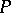. The minimal polynomial, the degree overand the conjugate numbers overfor algebraic numbers overare defined in a similar manner. A root of a polynomial with coefficients that are algebraic numbers overis an algebraic number over.

An algebraic number of arbitrary degreedoes not necessarily exist over any field. For instance, only algebraic numbers of the first degree exist over the field of complex numbers: the numbers of the field themselves. A given algebraic number can be of different degrees with respect to different fields; thus, the numberis an algebraic number of the second degree, but it is of the first degree with respect to the field of complex numbers. The set of all algebraic numbers over a fieldforms a number field.

Algebraic numbers, and algebraic number fields, were first systematically studied by C.F. Gauss (Gaussian numbers of the form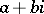, where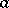and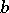are rational numbers). Gauss developed the arithmetic of Gaussian integers as a base for the theory of biquadratic residues. In their study of the theory of cubic residues, C.G.J. Jacobi and F.G. Eisenstein created the arithmetic of numbers of the form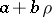, whereis a cubic root of unity, whileandare rational numbers. His attempts to produce a proof of the Fermat theorem led E. Kummer to conduct a deep study of cyclotomic fields (cf. Cyclotomic field), to introduce the concept of an ideal, and to create the elements of the theory of algebraic numbers. The theory of algebraic numbers was further developed by P. Dirichlet, L. Kronecker, D. Hilbert and others. Russian mathematicians — E.I. Zolotarev (theory of ideals), G.F. Voronoi (cubic irrationalities, units of cubic fields), A.A. Markov (cubic fields), Yu.V. Sokhotskii (theory of ideals) and others — also made significant contributions.

The concept of an algebraic number and the related concept of an algebraic number field are very important ideas in number theory and algebra. Algebraic numbers, which are a generalization of rational numbers, form subfields of algebraic numbers in the fields of real and complex numbers with special algebraic properties. The development of the theory of algebraic numbers greatly influenced the creation and development of the general theory of rings and fields.

Algebraic numbers have found numerous applications in various branches of number theory, algebra and other branches of mathematics: the theory of forms, Diophantine equations, Diophantine approximations, transcendental numbers, geometry of numbers, algebraic geometry, Galois theory, etc.

How to Cite This Entry:
Algebraic number. Encyclopedia of Mathematics. URL: http://encyclopediaofmath.org/index.php?title=Algebraic_number&oldid=17281
This article was adapted from an original article by A.B. Shidlovskii (originator), which appeared in Encyclopedia of Mathematics - ISBN 1402006098. See original article